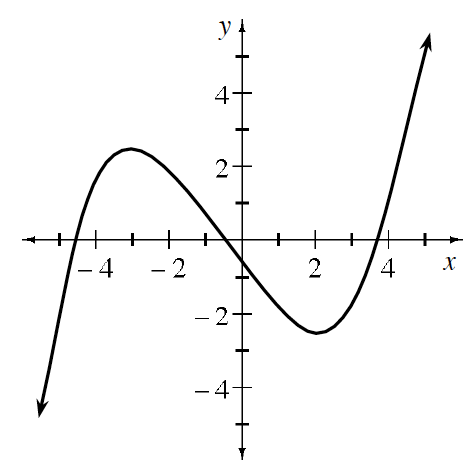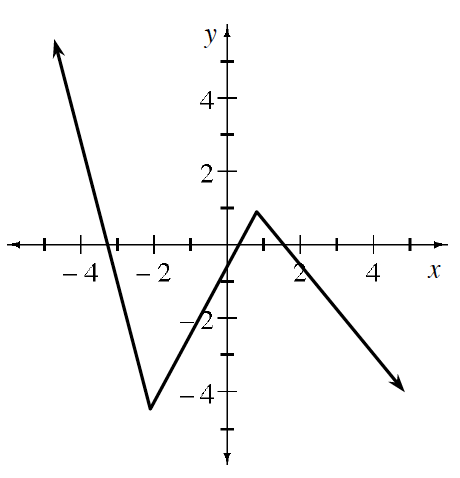### Home > CALC3RD > Chapter Ch1 > Lesson 1.3.3 > Problem1-132

1-132.

For each graph below, state the intervals where the function is increasing and decreasing. Homework Help ✎

 a.Hint (a):For which values of x is y increasing, and for which values of x is y decreasing?Answer (a):Increases when x < −3 and x >2, decreases when −3 < x < 2. b.Hint (b):See the hint in part (a).
1. For part (a) on the interval in which the function is decreasing, is the rate of decrease constant? How do you know?

Describe the shape of the graph where the function is decreasing? Is it curvy or linear? Is it steep or smooth or does it change?